Latest Banking jobs   »

# Quantitative Aptitude Quiz For RBI Grade B Phase 1 2023 – 09th May

Q1. Marked price of an article is Rs 360 more than its cost price. If profit earned is equal to the discount given then find the profit earned?
(a) Rs 190
(b) Rs 200
(c) Rs 150
(d) Rs 180
(e) Rs 175

Q2. Sudeep marked up the price at a price higher than cost price & gave 10% discount on every purchase and a complimentary chocolate box worth Rs. 300 on minimum purchase of Rs. 2000. This way he gained 20%. If a customer purchased for Rs. 3000. Find the cost price for Sudeep for the articles sold.
(a)Rs. 2500
(b) Rs. 2250
(c) Rs. 2750
(d)Rs. 2000
(e) None of these

Q3. Ashish buys a T.V at a discount of 15 %.If he buys the same T.V at a discount of 12.5%, then he would have to pay Rs. 175 more. Find the marked price of T.V?(in Rs.)
(a) 6000
(b) 7000
(c) 5000
(d)6500
(e) 7500

Q4. When a discount of 20% is given on a product then a loss of 4% occurs. When a discount of 6.5% is given on the same product, then what will be the profit percent?
(a) 13.4%
(b) 11.5%
(c) 16.9%
(d) 12.2%
(e) 15.8%

Q5. A shopkeeper marks up the price of a article by 40% above cost price. He gives 25% discount on marked price and earns Rs 420 profit. Find his profit if he give discount of 20% in place of 25%.
(a) Rs 1204
(b) Rs 1240
(c) Rs 1180
(d) Rs 1008
(e) Rs 1080

Q6. If a shopkeeper offers a single discount of 30% instead of two successive discounts of 25% and 12%, he loses Rs. 76 on selling an article. If the price of the article is marked up by 52% then find the cost price of that article.
(a) Rs. 1250
(b) Rs. 1200
(c) Rs. 1320
(d) Rs. 1650
(e) Rs. 1120

Q7. A shopkeeper marked the price of jeans 25% above and allowed two successive discounts of 10% and 5% respectively. If shopkeeper made a profit of Rs. 89.1, then find at what price shopkeeper sold the jeans to make a profit of 40%?
(a) 1844.4 Rs.
(b) 1814.4 Rs.
(c) 1860.4 Rs.
(d) 1890.4 Rs.
(e) 1880.4 Rs.

Q8. In mixture of milk and water in a jar, contains 72ℓ milk and 24ℓ water, Xℓ of milk and (X + 16) ℓ of water is added in mixture. If 12½% of new mixture measures 20ℓ, then find how much water was added in mixture ?
(a) 32 ℓ
(b) 28 ℓ
(c) 44 ℓ
(d) 40 ℓ
(e) 24 ℓ

Q9. In three containers A, B and C, A contains mixture of spirit and water in ratio 3 : 1 while B contains 20 L pure water and vessel C contains 30 L pure spirit .Half content of A is poured into B, and then content of B is poured into C. If content of C is poured again into A, then find initial quantity of mixture in A if final ratio of spirit and water in vessel A is 9 : 4.
(a) 80 L
(b) 60 L
(c) 75 L
(d) 92 L
(e) 65 L

Q10. A contains mixture of two liquids A and B in the ratio 5 : 3. If 8 litre of the mixture is taken out and replaced with B type liquid, then ratio of liquid A to liquid B in can become 1 : 1. Find the initial quantity of mixture in the Can.
(a) 40 L
(b) 30 L
(c) 35 L
(d) 24 L
(e) 48 L

Q11. The respective ratio of milk and water in the mixture is 4 : 3 respective. If 6 litre of water is added to this mixture, the respective ratio of milk and water becomes 8 : 7. Find the quantity of milk in the original mixture?
(a) 96 litre
(b) 36 litre
(c) 84 litre
(d) 48 litre
(e) 24 litre

Q12. A milkman adds 500 mℓ of water to each litre of milk in a container. He sells 30 ℓ of mixture from container and adds 10 litre milk in the remaining. The ratio of milk and water in the final mixture is 11 : 5. Find the initial quantity of milk in the container.
(a) 120ℓ
(b) 100 ℓ
(c) 160 ℓ
(d) 80 ℓ
(e) 125 ℓ

Q13. A container is filled with mixture of sodium, Potassium and chloride. Percentage of Potassium and Chloride are 40% and 25% respectively. If 20kg of mixture is taken out and 12kg of sodium and 15kg chloride is added to the container, then new ratio between sodium and chloride becomes 26: 25. Find the initial quantity of mixture in the container?
(a) 50kg
(b) 40kg
(c) 60kg
(d) 80kg
(e) 65kg

Q14. In first mixture, quantity of milk was 6 lit more than that of water. After adding 40 ltr of second mixture (having ratio of water to milk 3: 5) in the first mixture, ratio of water to milk in the final mixture becomes 9: 13. Find the total quantity of water in the final mixture.
(a) 27 ltr
(b) 31.5 ltr
(c) 36 ltr
(d) 45 ltr
(e) None of these

Q15. A container is full of milk, ¼th of the milk is taken out and is replaced with water, and this process is repeated 3 times and 297ℓ of milk is finally left in the container. find the capacity of container? (in ℓ)
(a) 528
(b) 644
(c) 740
(d) 704
(e) 750

Solutions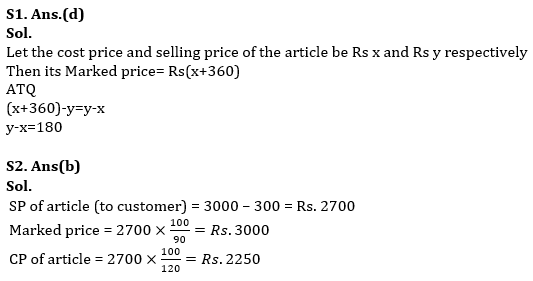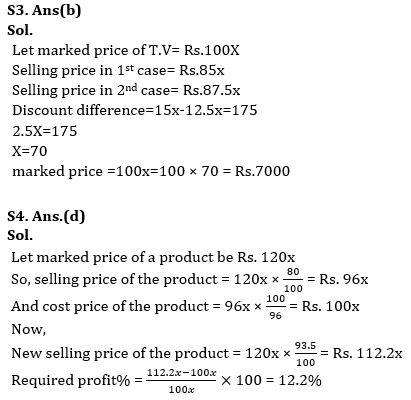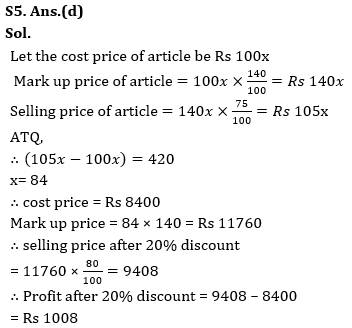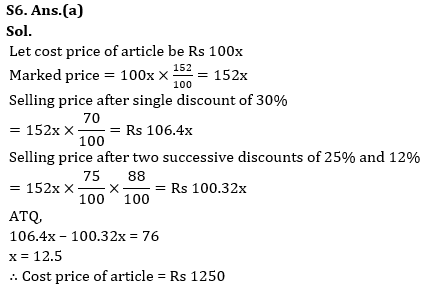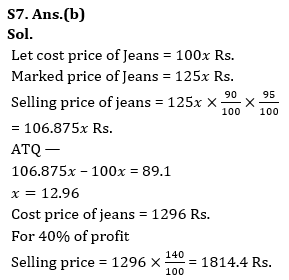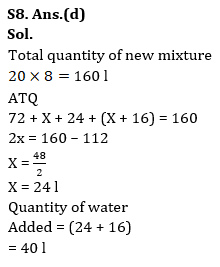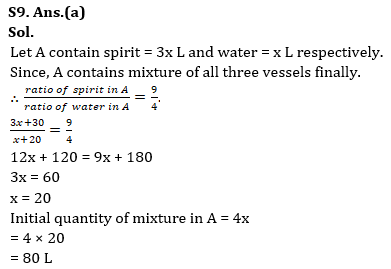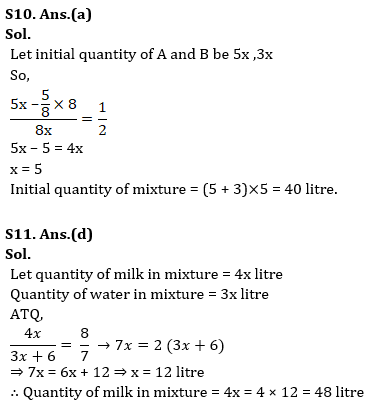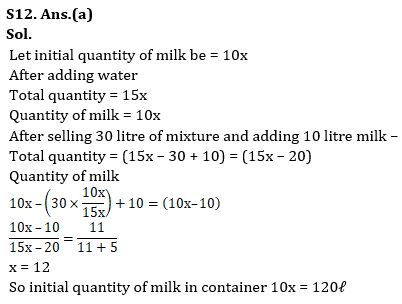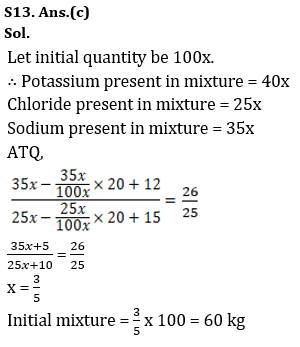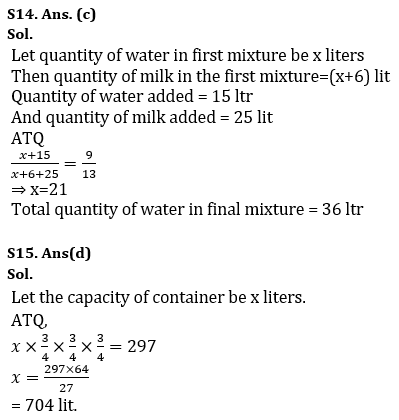## FAQs

### How many sections are there in the RBI Grade B Phase 1 Exam?

There are 4 sections in the RBI Grade B Phase 1 Exam i.e. English Language, General Awareness, Quantitative Aptitude & Reasoning.

#### Congratulations!Union Budget 2023-24: Free PDF